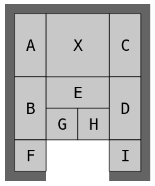CSES - HIIT Open 2017 - Klotski
• Time limit: 1.00 s
• Memory limit: 512 MB
A Klotski puzzle consists of a board of size $5 \times 4$ and $10$ blocks:
• $4$ blocks of size $2 \times 1$ (A, B, C, D)
• $1$ block of size $1 \times 2$ (E)
• $4$ blocks of size $1 \times 1$ (F, G, H, I)
• $1$ block of size $2 \times 2$ (X)
For example, here is one Klotski puzzle:On each move you can choose any block and move it one step left, right, up or down. The blocks must stay inside the board, and two blocks may never overlap. It is not allowed to rotate blocks.

Your goal is to move the $2 \times 2$ block to the position from which it can escape the board. What is the minimum number of moves needed for this?

Input

The input consists of five lines, each of which contains four characters. Each character is a letter (there is a block) or a dot (empty cell).

Output

Print one integer: the minimum number of moves needed to solve the puzzle. If the puzzle is unsolvable, output $-1$.

Example

Input:
AXXC AXXC BEED BGHD F..I

Output:
116